### Casino Probability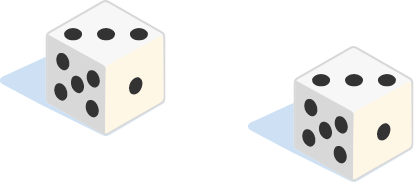When rolling a pair of standard six-sided dice, what is the probability that both dice land on the same number? (The illustration is just an example, any pair where the dice are the same will work.)

# Dice

Suppose you're playing a dice game against an opponent who is rolling two dice, whereas you are only rolling one die.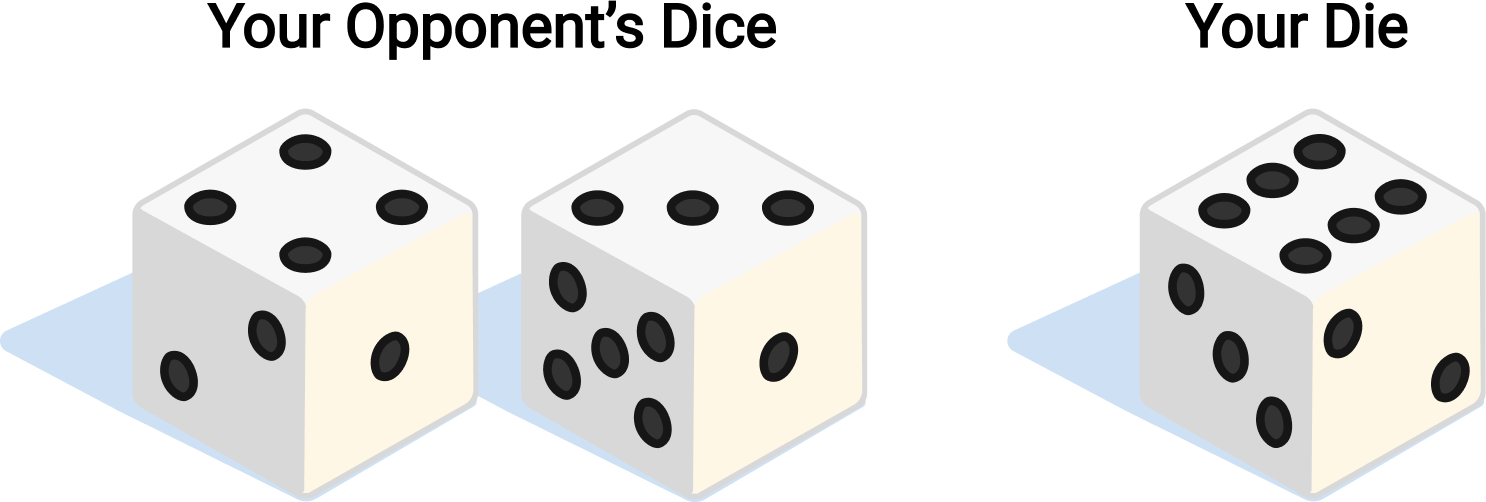Every time he rolls a sum of 7 on his two dice, he gives you a dollar. Every time you roll a 6 on your single die, you give him a dollar. If you both roll an equal number of times, is this a fair game (are you both equally likely to make money)?

# Dice

One essential tool for understanding casino games is expected value, the average of a value in a probability scenario over the long term. Suppose you play a game with a standard six-sided die which costs $\3$ each roll. You roll the die and then earn back the number on the top of the die: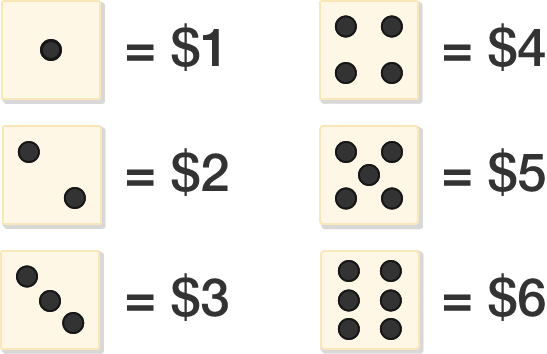We earn $1 for $\frac{1}{6}$ of the rolls,$2 for $\frac{1}{6}$ of the rolls, and so on, leading to an expected value per roll of

$\left(\frac{1}{6}\right)1 + \left(\frac{1}{6}\right)2 + \left(\frac{1}{6}\right)3 + \left(\frac{1}{6}\right)4 + \left(\frac{1}{6}\right)5 + \left(\frac{1}{6}\right)6 = 3.5.$

Since we are spending $3 a roll, this game will actually earn money for the player! # Dice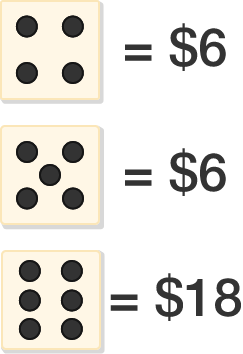Suppose you play a casino game with a standard six-sided die which costs$6 per roll. You earn nothing on a 1, 2, or 3; on a 4, 5, or 6 you earn as shown in the chart above.

Which is larger, the expected value you pay in per turn (so the casino earns money in the long term) or the expected value you win per turn (so you earn money in the long term)?

# Dice

Real casinos will always run games where they have an advantage (otherwise they wouldn't be in business too long!). However, it still can be true some bets are better than others.

Going back to the scenario with a casino game with a standard six-sided die, suppose you have two choices of bets:

• LOW: You earn $4 if the die comes up 1, 2, or 3, otherwise nothing. • SIX: You earn$10 if the die comes up 6, otherwise nothing.

Which bet will earn you more money in the long run?

# Dice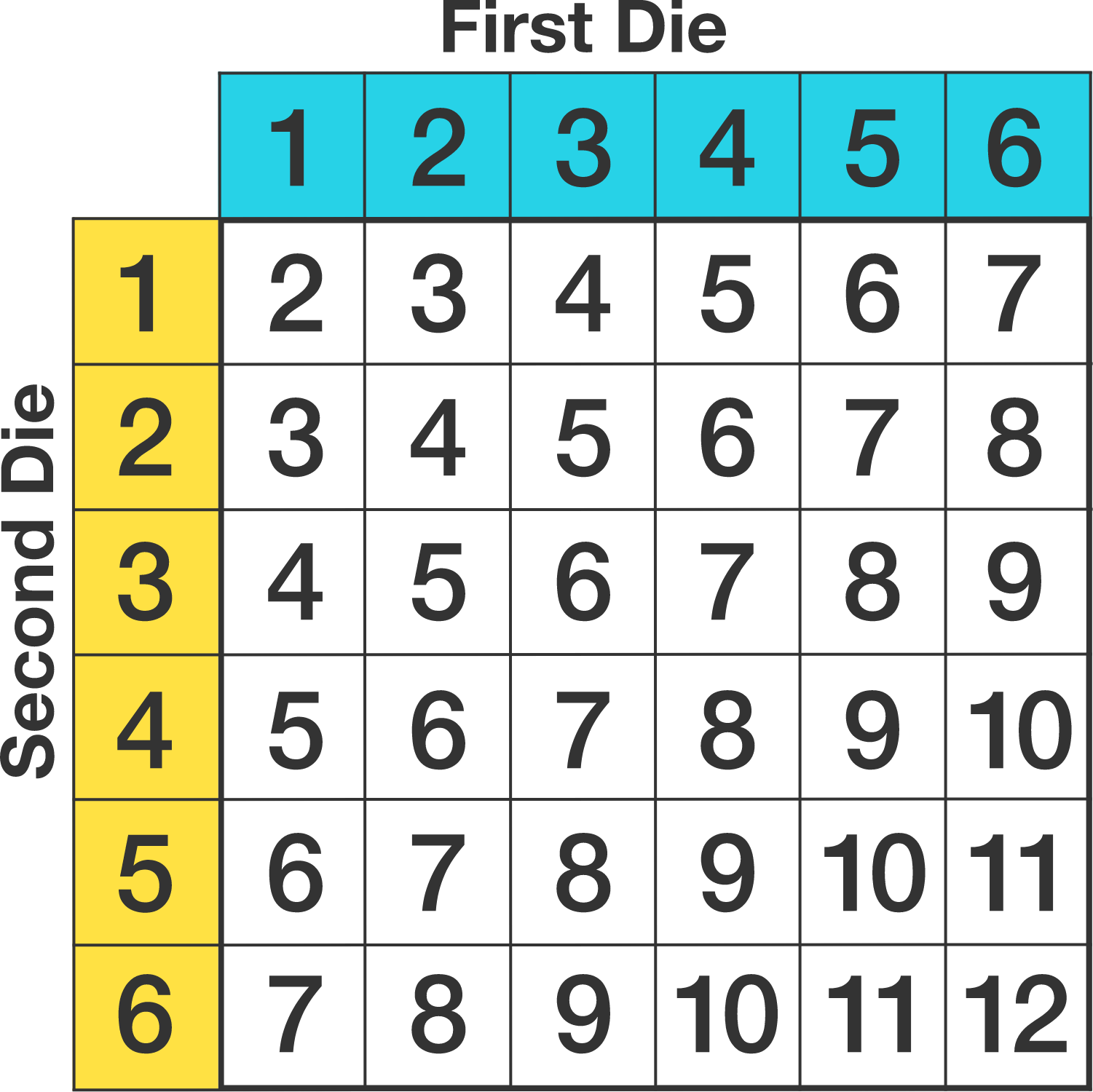Suppose someone plays a game with two standard dice with two bets based on the sum of the dice:

• SIX OR EIGHT: earns $1. • TWELVE: earns$10.

Which bet will earn the player more money in the long run?

×